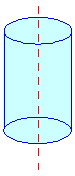Home MonkeyNotes Printable Notes Digital Library Study Guides Message Boards Study Smart Parents Tips College Planning Test Prep Fun Zone Help / FAQ How to Cite New Title Request

 8.4 Circular Cylinders Pillars, pipes etc. are examples of circular cylinders encountered daily. A circular cylinder is two circles with the same radius at a finite distance from each other with their circumferences joined.Figure 8.7 The definition of a circular cylinder is a prism with circular bases. The line joining the centers of the two circles is called the axis. If the axis is perpendicular to the circles it is a right circular cylinder otherwise it is an oblique circular cylinder. The lateral area of a right circular cylinder is the product of the circumference and the vertical distance between the two circles or the altitude ‘h’. = Circumference ´ h = 2pr ´ h = 2 p r h square units. where ’r’ is the radius of the circle. The total area must include apart from the lateral area, the areas of the two circles.         Area of the circle = pr2 \  Area of two circles = 2pr2 \         Total area       = 2prh + 2pr2                                   = 2pr (h + r)                                   = (h + r) square units where C = circumference. The volume of a right circular cylinder, = area of the circle ´ height = pr2 ´ h = pr2h cubic units. Index 8.1 Introduction to solid geometry 8.2 Prism 8.3 The cuboid and the cube 8.4 Cylinders 8.5 Pyramids 8.6 Right circular cone 8.7 Sphere Chapter 9
 Search: All Products Books Popular Music Classical Music Video DVD Toys & Games Electronics Software Tools & Hardware Outdoor Living Kitchen & Housewares Camera & Photo Cell Phones Keywords: